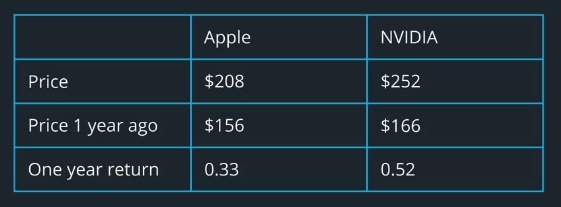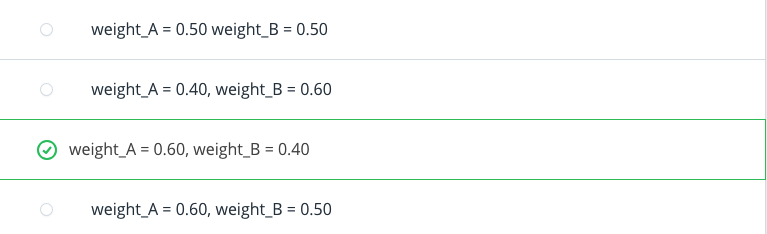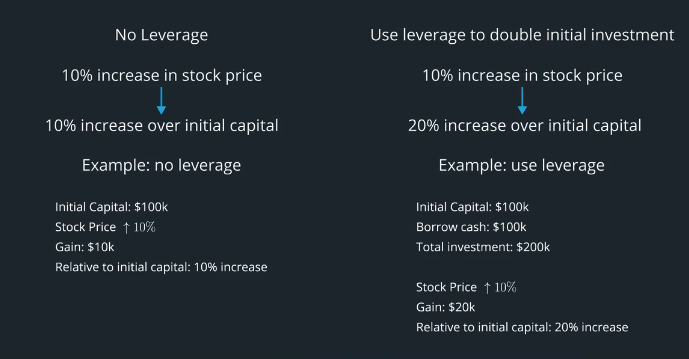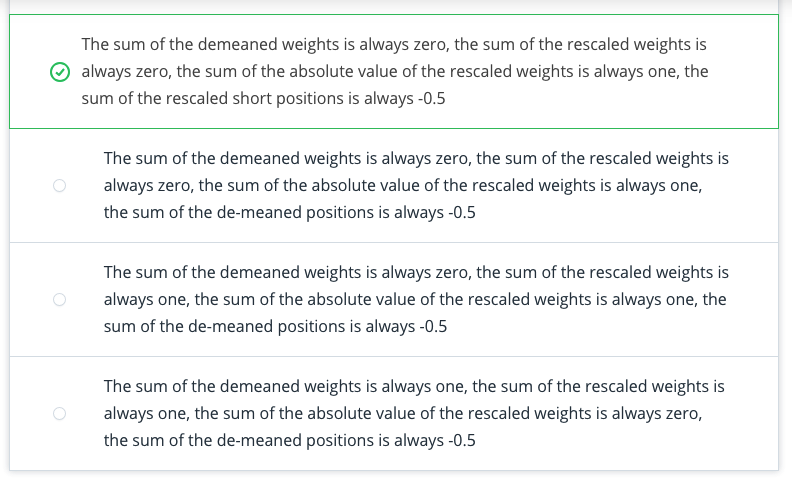# AI For Trading:Factors (49)

## What is a factor?

Factor: A list of numerical values, one for each stock, potentially predictive of an aspect of the performance
of these stocks in the fututer.

Factors can be based on:

• Momentum
• Fundamental information
• Signals from social media

Zipline: an open-source Python algorithmic trading library.

## Example of a factor

Hypothesis:one-year return indicates momentum for next few days.
Factor: one-year return of each stock in stock universe.## Quiz: factor values and weights

The factor value of stock A is higher than the factor value of stock B, and we use the factor value to determine portfolio weights for the stocks. Assume these are the only stocks in the portfolio, and that this is a long-only portfolio that invests all its cash in stocks, so the weights add up to 1. What are possible values for the weights of stock A and stock B?Do we want to put more money on stock A or more on stock B? What do the weights sum up to?
yes, weight_A should be greater than weight_B, and weights sum to 1.

## Standardizing a factor(标准化因子)

Now, let's see how to convert this raw calculation into a standardized factor## De-mean(去均值)

You just learned how to demean a factor so that the values add up to zero. But why do we do this.

### Notional(概念)

A portfolio's notional is the number we can multiply the stock weights by in order to get a dollar value for each stock's position. For a long-only portfolio, we can think of this as the amount of cash that a fund has available to invest in the portfolio. Whether the positions are long or short, we can multiply the stock weight to the notional to turn this into a dollar amount for that stock's position.

## Rescale

### Leverage(杠杆作用)

Leverage is the act of borrowing in order to invest. Borrowing cash in order to go long on more positions may magnify gains but also losses.### Leverage Ratio(杠杆率）

The leverage ratio is the sum of the magnitudes of all positions, divided by the notional. The leverage ratio gives a sense of how much risk a portfolio is taking, because taking more positions magnifies both gains and losses.

To standardize a factor, we divide by the sum of the magnitudes (sum of the absolute value of the positions), so that this rescaled vector's sum of magnitudes is equal to one. This makes different factors more comparable, because it's as if you're comparing different portfolios but each with the same amount of money placed on their positions.

## Standardizing a Factor

To make a factor dollar neutral, subtract its mean from each position. To make the factor have a leverage ratio of one, divide by the sum of the absolute value of the positions.

## Quiz:dollar neutral and leverage ratio

Choose factor values (any numbers) for three stocks. De-mean these stocks and make a note of their sum. Then rescale the demeaned values so that the sum of their absolute values equals one. Make a note of their sum. Change the starting factor values a few times. What do you notice?The sum of the demeaned weights is always zero, the sum of the rescaled weights is always zero, the sum of the absolute value of the rescaled weights is always one, the sum of the rescaled short positions is always -0.5